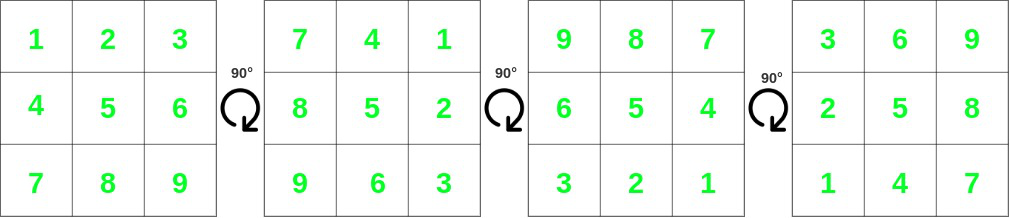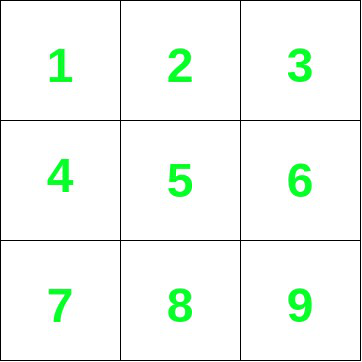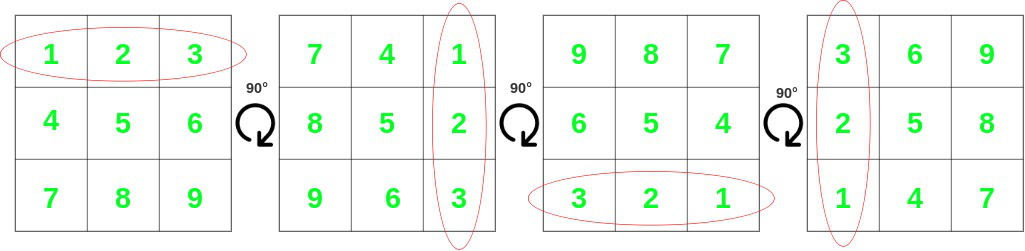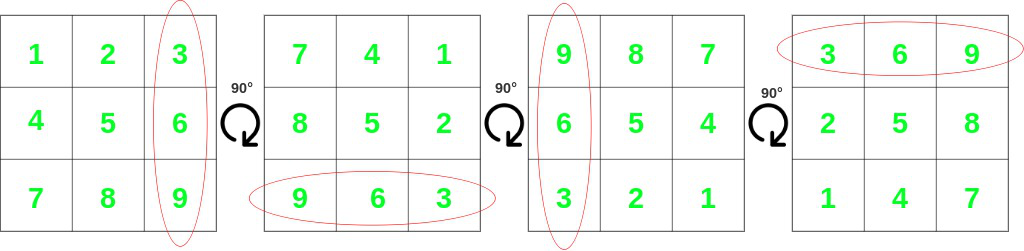Check whether Matrix T is a result of one or more 90° rotations of Matrix mat

• Difficulty Level : Hard
• Last Updated : 29 Jun, 2021

Given two 2D matrices mat[][] and T[][] of size M×N and P×Q respectively. The task is to check whether the matrix T[][] is a result of one or more 90° rotations of the matrix mat[][].

Examples:

Input: mat[][] = {{1, 2, 3}, {4, 5, 6}, {7, 8, 9}}, T[][] ={{7, 4, 1}, {8, 5, 2}, {9, 6, 3}}
Output: Yes
Explanation:From the figures given above, it is clear that T[][] is obtained by rotating 90° once.

Input: mat[][] = {{1, 2, 3}, {4, 5, 6}, {7, 8, 9}}, T[][] = {{1, 4, 7}, {8, 5, 2}, {9, 6, 3}}
Output: No

Approach: The given problem can be solved based on the following observations:

• Consider the following 2D matrix:• Rotating it several times by 90° gets the following matrices:• From the above figures, it can be seen that every row can either occur as it is or in its reversed form.• The same can be observed for the columnsHence, if T[][] is one of the rotated forms of mat[][], it must have at least one occurrence of the original or the reversed form of mat[][]’s rows and columns present as its rows and columns.

Follow the steps below to solve the problem:

• If the dimensions of mat[][] and T[][] are not equal or not then print “No” and return.
• Initialize a map of vectors, say m to store the frequencies of all rows, columns, and their reversed versions.
• Iterate in the range [0, M-1] and using the variable i and perform the following steps:
• Increment m[mat[i]] by 1 and then reverse the vector mat[i] and then increment m[mat[i]] by 1.
• Iterate in the range [0, N-1] and using the variable i and perform the following steps:
• Push all the elements of the ith column in a vector say r.
• Increment m[r] by 1 and then reverse the vector r and then increment m[r] by 1.
• Iterate in the range [0, M-1] and using the variable i and perform the following steps:
• If m[T[i]] is less than 0 then print “No” and return.
• Otherwise, decrement m[T[i]] by 1.
• Iterate in the range [0, N-1] and using the variable i and perform the following steps:
• Push all the elements of the ith column of T[][] in a vector say r.
• If m[r] is less than 0 then print “No” and return.
• Otherwise, decrement m[r] by 1.
• Finally, if none of the above cases satisfy then print “Yes“.

Below is the implementation of the above approach:

C++

 // C++ program for the above approach #include using namespace std;   // Function to check whether another // matrix can be created by rotating // mat one or more times by 90 degrees string findRotation(vector >& mat,                     vector >& T) {     // If the dimensions of both the     // arrays don't match     if (T.size() != mat.size()         || T.size() != mat.size()) {         // Return false         return "No";     }       // Map to store all rows, columns     // and their reversed versions     map, int> m;       // Iterate in the range [0, M-1]     for (int i = 0; i < mat.size(); i++) {           // Increment the frequency of the         // i'th row by 1         m[mat[i]] += 1;           // Reverse the i'th row         reverse(mat[i].begin(), mat[i].end());           // Increment the frequency of the         // i'th row by 1         m[mat[i]] += 1;     }       // Iterate in the range [0, N-1]     for (int i = 0; i < mat.size(); i++) {           // Stores the i'th column         vector r = {};           // Iterate in the range [0, M-1]         for (int j = 0; j < mat.size(); j++) {             r.push_back(mat[j][i]);         }           // Increment the frequency of the         // i'th column by 1         m[r] += 1;           // Reverse the i'th column         reverse(r.begin(), r.end());           // Increment the frequency of the         // i'th column by 1         m[r] += 1;     }       // Iterate in the range [0, M-1]     for (int i = 0; i < T.size(); i++) {           // If frequency of the i'th row         // is more in T[][] than in the         // mat[][].         if (m[T[i]] <= 0) {             return "No";         }           // Decrement the frequency of the         // i'th row by 1         m[T[i]] -= 1;     }       // Iterate in the range [0, N-1]     for (int i = 0; i < T.size(); i++) {           // Stores the ith column         vector r = {};           // Iterate in the range [0, M-1]         for (int j = 0; j < T.size(); j++) {             r.push_back(T[j][i]);         }           // If frequency of the i'th column         // is more in T[][] than in mat[][].         if (m[r] <= 0) {             return "No";         }           // Decrement the frequency of the i'th         // column by 1         m[r] -= 1;     }       // Return "Yes"     return "Yes"; }   // Driver code int main() {     // Input     vector > mat         = { { 1, 2, 3 }, { 4, 5, 6 }, { 7, 8, 9 } };     vector > T         = { { 3, 6, 9 }, { 2, 5, 8 }, { 1, 4, 7 } };       // Function call     cout << findRotation(mat, T);       return 0; }

Javascript



Output

Yes

Time Complexity: O(N*M)
Auxiliary Space: (N*M)

My Personal Notes arrow_drop_up
Recommended Articles
Page :